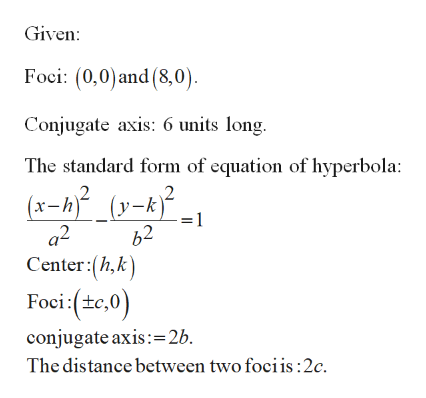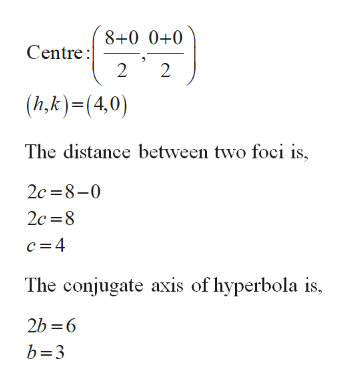# Find the standard form for the equation of the hyperbola with foci (0,0) and (8,0) if the conjugate axis is 6 units long.

Question
14 views

Find the standard form for the equation of the hyperbola with foci (0,0) and (8,0) if the conjugate axis is 6 units long.

check_circle

Step 1

Standard form of equation of hyperbola.help_outlineImage TranscriptioncloseGiven: Foci: (0,0)and (8,0). Conjugate axis: 6 units long. The standard form of equation of hyperbola: (x-h)² (y-k) 1 62 Center:(h,k) a2 Foci:(tc,0) conjugate axis:=2b. The distance between two fociis:2c. fullscreen
Step 2

Find center:

Take midpoin...help_outlineImage Transcriptionclose8+0 0+0 Centre : 2 (h,k)=(4,0) The distance between two foci is, 2c =8-0 2c =8 c= 4 The conjugate axis of hyperbola is, 2b =6 b=3 fullscreen

### Want to see the full answer?

See Solution

#### Want to see this answer and more?

Solutions are written by subject experts who are available 24/7. Questions are typically answered within 1 hour.*

See Solution
*Response times may vary by subject and question.
Tagged in

### Geometry